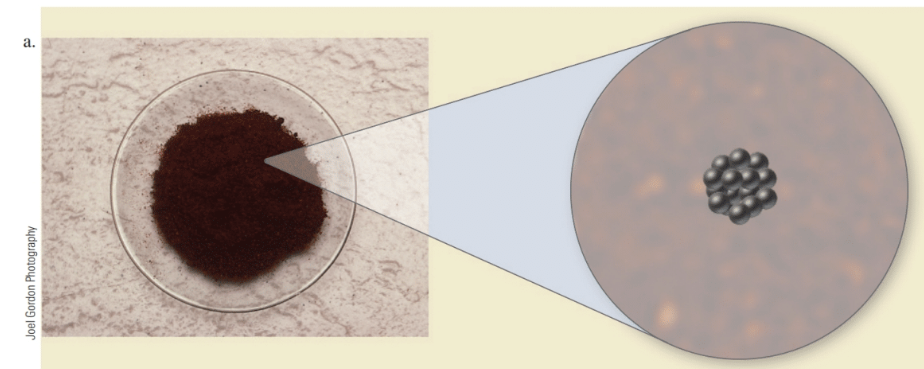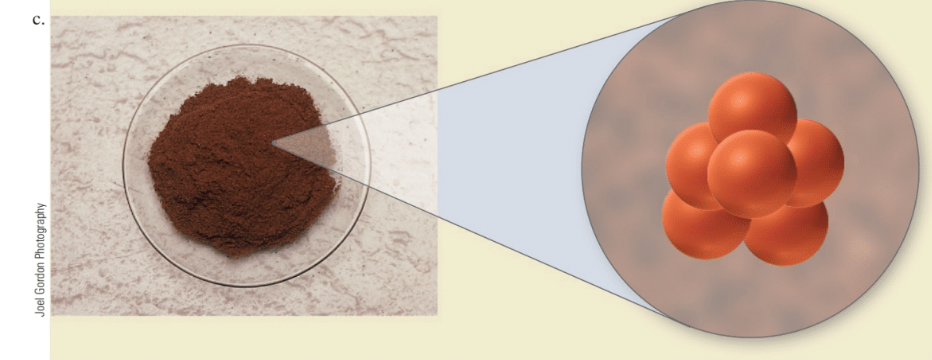# Here are three fictitious elements and a molecular view of the atoms that compose them. The molar mass of the middle element, (b), is 25 grams per dozen (g/doz). (The atoms of these fictitious elements are much larger than ordinary atoms.) Based on the size of the atoms, do you expect the atomic masses of elements (a) and (c) to be greater than or less than (b)? How many atoms are present in 175 g of element (b)?### Chemistry In Focus

7th Edition
Tro + 1 other
Publisher: Cengage Learning,
ISBN: 9781337399692

#### Solutions

Chapter
Section### Chemistry In Focus

7th Edition
Tro + 1 other
Publisher: Cengage Learning,
ISBN: 9781337399692
Chapter 3, Problem 65E
Textbook Problem
26 views

## Here are three fictitious elements and a molecular view of the atoms that compose them. The molar mass of the middle element, (b), is 25 grams per dozen (g/doz). (The atoms of these fictitious elements are much larger than ordinary atoms.)Based on the size of the atoms, do you expect the atomic masses of elements (a) and (c) to be greater than or less than (b)? How many atoms are present in 175 g of element (b)?

Interpretation Introduction

Interpretation:

The atomic masses of three fictitious elements are to be compared, and the number of atoms present in a given mass is to be calculated.

Concept Introduction:

 A mole is a unit of amount of substance. It relates the number of particles to the molar mass.

 One mole of a substance contains 6.022×1023 (Avogadro’s number) particles. The number remains constant regardless of the nature of the substance. One mole of an atom contains 6.022×1023 atoms. One mole of molecules contains 6.022×1023 molecules.

 It is also related to the molar mass of a substance in grams. One mole of a substance weighs that amount in grams which is equal to its molar mass.

 To sum up, 1 mole of a substance contains 6.022×1023 particles and weighs equal to its molar mass in grams.

 One dozen equals twelve units of the same type.

### Explanation of Solution

As per the figures, atoms of (c) have greater atomic mass than atoms of (b). So, the atomic mass of (c) is more than that of (b).

Atoms of (a) have smaller atomic mass than atoms of (b). So, the atomic mass of (a) is less than that of (b).

The number of atoms present in element (b) is calculated as follows:

Atomic mass of element (b) = 25 g/dozen (given)25 g of element (b) = 1 dozen175 g of element (b) = 1 dozen25g×175

### Still sussing out bartleby?

Check out a sample textbook solution.

See a sample solution

#### The Solution to Your Study Problems

Bartleby provides explanations to thousands of textbook problems written by our experts, many with advanced degrees!

Get Started

Find more solutions based on key concepts
Which diet has been shown to increase an athlete's endurance? high-fat diet normal mixed diet high-carbohydrate...

Nutrition: Concepts and Controversies - Standalone book (MindTap Course List)

Why don’t red dwarfs become giant stars?

Horizons: Exploring the Universe (MindTap Course List)

What is genomics?

Human Heredity: Principles and Issues (MindTap Course List)

Why are buffers important for living organisms?

Biology: The Dynamic Science (MindTap Course List)

A 6.00-V battery is connected across the primary coil of a transformer having 50 turns. If the secondary coil o...

Physics for Scientists and Engineers, Technology Update (No access codes included)

Translate the .sequence of bases in the previous question, starting at the second base.

Biology: The Unity and Diversity of Life (MindTap Course List)

How is a seal different from a sea lion? Which animal is featured in seal shows at oceanaria?

Oceanography: An Invitation To Marine Science, Loose-leaf Versin